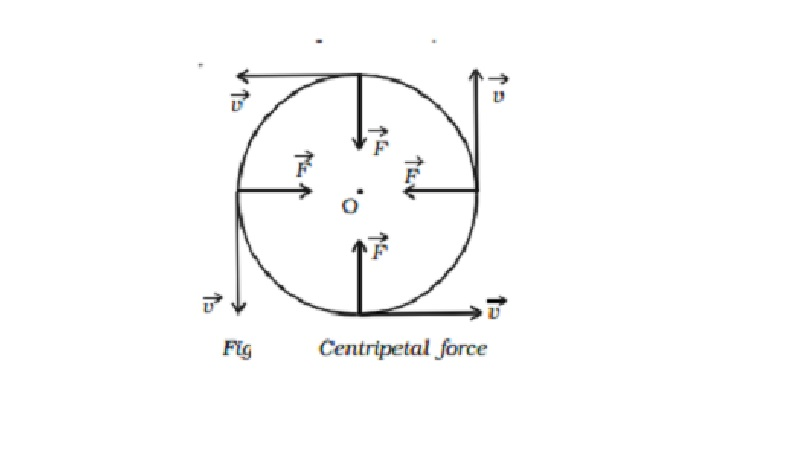Home | | Physics | | Physics | Centripetal acceleration, Centripetal force and Centripetal reaction

# Centripetal acceleration, Centripetal force and Centripetal reactionAccording to Newton?s first law of motion, a body possesses the property called directional inertia (i.e) the inability of the body to change its direction. This means that without the application of an external force, the direction of motion can not be changed.

Centripetal acceleration

The speed of a particle performing uniform circular motion remains constant throughout the motion but its velocity changes continuously due to the change in direction (i.e) the particle executing uniform circular motion is said to possess an acceleration.

Consider a particle executing circular motion of radius r with linear velocity v and angular velocity ω. The linear velocity of the particle acts along the tangential line. Let dθ be the angle described by the particle at the centre when it moves from A to B in time dt.

At A and B, linear velocity v acts along AH and BT respectively. In Fig.   AOB = HET ( angle subtended by the two radii of a circle = angle subtended by the two tangents).

The velocity v at B of the particle makes an angle dθ with the line BC and hence it is resolved horizontally as v cos dθ along BC and vertically as v sin d θ along BD.

The change in velocity along the horizontal direction = v cos dθ -v

If dθ is very small, cos dθ = 1

Change in velocity along the horizontal direction = v �' v = 0

(i.e) there is no change in velocity in the horizontal direction.

The change in velocity in the vertical direction (i.e along AO) is

dv = v sin dθ �' 0 = v sin dθ

If dθ is very small, sin dθ = dθ

The change in velocity in the vertical direction (i.e) along radius of the circledv = v.dθ ...(1)

But, acceleration a = dv/dt = v dθ/dt = vω ...(2)

where ω = dθ/dt is the angular velocity of the particle.

We know that v = r ω ...(3)

From equations (2) and (3),

a = rω ω = rω2 = v2/r ...(4)

Hence, the acceleration of the particle producing uniform circular motion is equal to  v2/r and is along AO (i.e) directed towards the centre of the circle. This acceleration is directed towards the centre of the circle along the radius and perpendicular to the velocity of the particle. This acceleration is known as centripetal or radial or normal acceleration.

Centripetal force

According to Newton's first law of motion, a body possesses the property called directional inertia (i.e) the inability of the body to change its direction. This means that without the application of an external force, the direction of motion can not be changed. Thus when a body is moving along a circular path, some force must be acting upon it, which continuously changes the body from its straight-line path (Fig). It makes clear that the applied force should have no component in the direction of the motion of the body or the force must act at every point perpendicular to the direction of motion of the body. This force, therefore, must act along the radius and should be directed towards the centre.Hence for circular motion, a constant force should act on the body, along the radius towards the centre and perpendicular to the velocity of the body. This force is known as centripetal force.

If m is the mass of the body, then the magnitude of the centripetal force is given by

F       = mass x centripetal acceleration

=m (rω2)Examples

Any force like gravitational force, frictional force, electric force, magnetic force etc. may act as a centripetal force. Some of the examples of centripetal force are :

i.   In the case of a stone tied to the end of a string whirled in a circular path, the centripetal force is provided by the tension in the string.

ii.      When a car takes a turn on the road, the frictional force between the tyres and the road provides the centripetal force.

iii.       In the case of planets revolving round the Sun or the moon revolving round the earth, the centripetal force is provided by the gravitational force of attraction between them

iv.       For an electron revolving round the nucleus in a circular path, the electrostatic force of attraction between the electron and the nucleus provides the necessary centripetal force.

Centrifugal reaction

According to Newton's third law of motion, for every action there is an equal and opposite reaction. The equal and opposite reaction to the centripetal force is called centrifugal reaction, because it tends to take the body away from the centre. In fact, the centrifugal reaction is a pseudo or apparent force, acts or assumed to act because of the acceleration of the rotating body.

In the case of a stone tied to the end of the string is whirled in a circular path, not only the stone is acted upon by a force (centripetal force) along the string towards the centre, but the stone also exerts an equal and opposite force on the hand (centrifugal force) away from the centre, along the string. On releasing the string, the tension disappears and the stone flies off tangentially to the circular path along a straight line as enuciated by Newton's first law of motion.

When a car is turning round a corner, the person sitting inside the car experiences an outward force. It is because of the fact that no centripetal force is supplied by the person. Therefore, to avoid the outward force, the person should exert an inward force.

Study Material, Lecturing Notes, Assignment, Reference, Wiki description explanation, brief detail

Related Topics